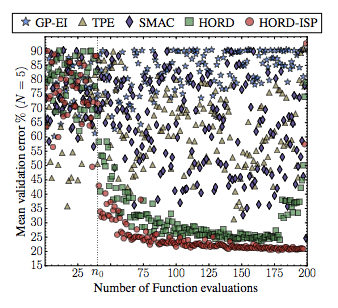# d347: Efficient Deep Learning Hyperparameter Optimization Using Deterministic RBF Surrogates

[AAAI2017 Paper] Efficient Deep Learning Hyperparameter Optimization: https://arxiv.org/pdf/1607.08316v2.pdfAbstract: “Automatically searching for optimal hyperparameter configurations is of crucial importance for applying deep learning algorithms in practice. Recently, Bayesian optimization has been proposed for optimizing hyperparameters of various machine learning algorithms. Those methods adopt probabilistic surrogate models like Gaussian processes to approximate and minimize the validation error function of hyperparameter values. However, probabilistic surrogates require accurate estimates of sufficient statistics (e.g., covariance) of the error distribution and thus need many function evaluations with a sizeable number of hyperparameters. This makes them inefficient for optimizing hyperparameters of deep learning algorithms, which are highly expensive to evaluate. In this work, we propose a new deterministic and efficient deep learning hyperparameter optimization method that employs radial basis functions as error surrogates. The proposed mixed integer algorithm, called HORD, searches the surrogate for the most promising hyperparameter values through dynamic coordinate search and requires many fewer function evaluations. HORD does well in low dimensions but it is exceptionally better in higher dimensions. Extensive evaluations on MNIST and CIFAR-10 for four deep neural networks demonstrate HORD significantly outperforms the well-established Bayesian optimization methods such as GP, SMAC, and TPE. For instance, on average, HORD is more than 6 times faster than GP-EI in obtaining the best configuration of 19 hyperparameters.